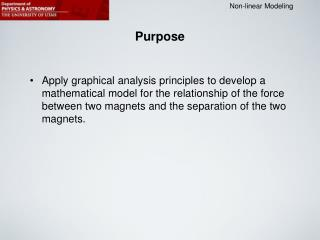DownloadDownload PresentationPurpose

# Purpose

Télécharger la présentation## Purpose

- - - - - - - - - - - - - - - - - - - - - - - - - - - E N D - - - - - - - - - - - - - - - - - - - - - - - - - - -
##### Presentation Transcript

1. Purpose • Apply graphical analysis principles to develop a mathematical model for the relationship of the force between two magnets and the separation of the two magnets.

2. A Good Guess of the Force Law a number a constant Force between the two magnets Distance between the two magnets We can easily find/imagine that the force between two magnets is less when they are separated further apart (larger r).  We expect n < 0 • Basic procedure: • Measure F for different r. • Plot F versus r in Excel. • Create a trendline using the Power trend/regression option. • Use log-log plot to determine how n varies with r.

3. Position of the Large Magnet Initial separation of the two magnets. r  5mm Initial position of large magnet: Clamp at low end of magnet. Then: Increase r by moving the large magnet but leaving the clamp in the same position.

4. The Polarity of the Magnets Blue and red colors indicate the polarity of the magnets as they face each other in the setup: - Two “blue” or two “red” magnets will repel each other. - Two unequally colored magnets will attract each other. You may need to borrow a red or blue small magnet from another group to do both attractive and repulsive force measurements.

5. Finding the Exponent Using a Log-Log Plot In this example (varying r): slope = n log F slope of log F versus log r plot log r